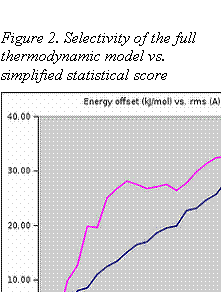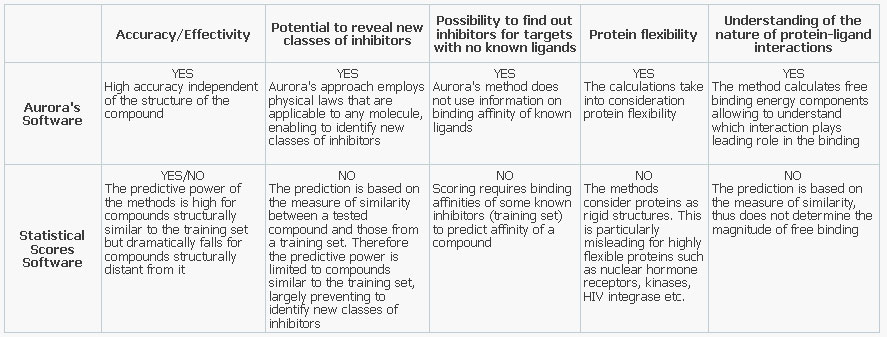We produce and sell unique chemicals around the world

# Quantum and Molecular Physics

## Aurora Drug Discovery Software

Aurora employs quantum mechanics, thermodynamics, and an advanced continuous water model for solvation effects to calculate ligand´s binding affinities. This approach differs dramatically from scoring functions that are commonly used for binding affinity predictions. By including the entropy and aqueous electrostatic contributions in to the calculations directly, Aurora algorithms  produce much more accurate and robust values of binding free energies.

Interaction of a ligand with a protein is characterized by the value of binding free energy. The free energy (F) is the thermodynamic quantity that is directly related to experimentally measurable value of inhibition constant (IC50) and depends on electrostatic, quantum, aqueous solvation forces, as well as on statistical properties of interacting molecules. There are two major contributing quantities leading to non-additivity in F: 1) the electrostatic and solvation energy and 2) the entropy.Most of the popular scores (e.g. AutoDock, Dock 5, XSCORE, GOLD, etc.) employ a reasonable approximation for short-range quantum interactions but do not perform a detailed calculation of aqueous electrostatics and entropy. Both the solvation energy and the entropy are difficult to compute: so instead of exact computations scoring functions use an approximation. In this approximation the contributions of non-additive properties are estimated as fractions of easier-to-calculate pairwise interactions: electrostatics and van der Waals forces.

Such approximations work to some extent because vacuum electrostatics is nearly canceled by solvation energies; at the same time the enthalpy of binding is approximately compensated by entropy. Therefore, the calculations of solvation energies and entropy seemingly can be avoided by combining molecular mechanics, van der Waals and electrostatic forces linearly, usually with small  numerical coefficients (of the order of 10%).

However, a potential energy surface given by such linear combinations of unrelated quantities with statistics-based coefficients is not necessarily related to the true interaction profile. That is why such a simple score fails frequently to reproduce unique binding modes and hence contributes to docking false negatives. In the same time, this approximation tends to overestimate the affinity of weak binders producing docking false positives. Thus, in spite of reasonable accuracy of such predictions the selectivity of scoring function is low. This means that frequently scoring functions will not allow to identify a really strong binder among the pool of similar weak binders. Moreover, affinities of weak binders may be overestimated.

Aurora software does not rely on approximate cancellations of important physical quantities. Instead, we employ our original continuous water model to compute both the vacuum and aqueous electrostatic energies, quantum mechanics to calculate the short range forces and thermodynamic sampling to obtain the value of the free energy (entropy). As a result we can not only observe the necessary subtractions of individual energy component, but also perform molecular modeling in a more realistic and physically justified way. Fig. 1 shows the results of a docking run on a single rigid protein structure for 220 different ligands. R.m.s. error in free energy is 2kJ/mol, the correlation coefficient is 0.7.The selectivity improvement in our approach is illustrated by the following model calculation. First, we derived a simple linear model directly from our Aurora vacuum force field. The short range interactions were adjusted with variations (to allow for empirical hydrogen bonds). The  van der Waals “scaling factors” and “protein dielectric constant” were found by correlating the suggested score with experimental binding affinities for a number of known complexes. Both the linear score and complete Aurora force field were tested using 300 protein-ligand pairs and showed comparable accuracy.

Fig. 2 shows docking funnels (the energy difference between the conformers plotted as a function of r.m.s. from the known crystallographic position of a ligand). Both full Aurora free energies (red lines) and scoring function (blue lines) were used to calculate, in the case of Aurora or estimate, in the case of linear score, the free energies for numerous binding modes (conformers). The conformers were generated by the Aurora docking program. The resulting energies were averaged over the conformers with similar r.m.s. values and plotted on the same graph.

The model calculation shows that the Aurora force field has a much steeper docking funnel, i.e. is more sensitive to misplacements of a ligand, than a scoring function. Therefore, the Aurora complete force field can be used to distinguish similar binding modes and hence obtain much more accurate docking positions.  As a result, Aurora shows a dramatically lower ratio of false positives and false negatives as compared with a scoring function based method.

In fact, non-additive interactions (especially solvation effects) play a key role in the molecular recognition of small molecules by proteins. Aurora’s approach is practically the only accurate and highly sensitive method available to a broad audience of researchers, which is able to realistically model intermolecular interactions.

Table shows some advantages of Aurora's software compared to the known statistical scores software: